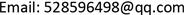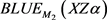﻿ 两线性随机效应模型未知参数函数预测量的等价性研究 Equivalence Study of Unknown Parameter Function Predictors under Two Linear Random Effects Models

Vol. 08  No. 10 ( 2019 ), Article ID: 32652 , 9 pages
10.12677/AAM.2019.810188

Equivalence Study of Unknown Parameter Function Predictors under Two Linear Random Effects Models

Ya Cai, Zhuqiu Zhang, Yiqin Ye

School of Data Science and Information Engineering, Guizhou Minzu University, Guiyang GuizhouReceived: Oct. 1st, 2019; accepted: Oct. 17th, 2019; published: Oct. 24th, 2019ABSTRACT

Suppose that adding a new variable term to the general linear random-effect model becomes the overparameter linear random-effect model, then the statistical inference of the some unknown parameter of the two models is not necessarily the same. In order to solve this problem, this paper uses the solution of constrained quadratic matrix-valued function optimization problem to give the analytical expression of the best linear unbiased predictor/best linear unbiased estimator of the unknown parameter function under the overparameter linear random-effect model. The conditions for the equivalence of the best linear unbiased predictor/best linear unbiased estimator of unknown parameter functions of two models are obtained by using some algebra and matrix theory tools.

Keywords:Linear Random-Effect Model, Best Linear Unbiased Predictor, Best Linear Unbiased Estimator, Covariance Matrix, Equivalence1. 引言

2. 模型与方法

${M}_{1}:\text{ }\text{ }\text{ }\text{ }\text{ }y=X\beta +\epsilon ,\text{ }\text{ }\beta =Z\alpha +\gamma$ (1)

${M}_{2}:\text{ }\text{ }\text{ }\text{ }\text{ }\text{ }\text{ }y=X\beta +F\zeta +\epsilon ,\text{ }\text{ }\beta =Z\alpha +\gamma$ (2)

$E\left[\begin{array}{l}\gamma \\ \epsilon \end{array}\right]=0$ , $Cov\left[\begin{array}{l}\gamma \\ \epsilon \end{array}\right]=\left[\begin{array}{cc}{\Sigma }_{11}& {\Sigma }_{12}\\ {\Sigma }_{21}& {\Sigma }_{22}\end{array}\right]=\Sigma$ (3)

$D\left(y\right)=D\left(X\gamma +\epsilon \right)=X{\Sigma }_{11}{X}^{\prime }+X{\Sigma }_{12}+{\Sigma }_{21}{X}^{\prime }+{\Sigma }_{22}=\stackrel{˜}{X}\Sigma {\stackrel{˜}{X}}^{\prime }$ (4)

$Cov\left(X\gamma ,y\right)=Cov\left(X\gamma ,X\gamma +\epsilon \right)=X{\Sigma }_{11}{X}^{\prime }+{\Sigma }_{12}=X\Sigma {\stackrel{˜}{X}}^{\prime }$ (5)(6)

$\varphi =A\alpha +B\gamma +C\epsilon$ (7)

$f\left({L}_{u}\right)=\left({L}_{u}{C}_{1}+{D}_{1}\right)M{\left({L}_{u}{C}_{1}+{D}_{1}\right)}^{\prime }\text{ }\text{ }\text{ }\text{ }\text{ }\text{ }\text{s}\text{.t}\text{ }\text{ }\text{ }\text{ }\text{ }{L}_{u}{A}_{1}={B}_{1}$

$f\left({L}_{u}\right)\ge f\left(L0\right)$

${L}_{0}\left[{A}_{1},{C}_{1}M{{C}^{\prime }}_{1}{A}_{1}^{\perp }\right]=\left[{B}_{1},-{D}_{1}M{{C}^{\prime }}_{1}{A}_{1}^{\perp }\right]$

${L}_{0}$ 的一般表达式及相应地 $f\left({L}_{0}\right)$$f\left({L}_{u}\right)-f\left({L}_{0}\right)$ 如下

${L}_{0}=\mathrm{arg}\underset{{L}_{u}{A}_{1}={B}_{1}}{\mathrm{min}}f\left({L}_{u}\right)=\left[{B}_{1},-{D}_{1}M{{C}^{\prime }}_{1}{A}_{1}^{\perp }\right]{\left[{A}_{1},{C}_{1}M{{C}^{\prime }}_{1}{A}_{1}^{\perp }\right]}^{+}+U{\left[{A}_{1},{C}_{1}M{{C}^{\prime }}_{1}\right]}^{\perp }$

$f\left({L}_{0}\right)=\underset{{L}_{u}{A}_{1}={B}_{1}}{\mathrm{min}}f\left({L}_{u}\right)=KM{K}^{\prime }-KM{C}_{1}T{{C}^{\prime }}_{1}M{K}^{\prime }$

$\begin{array}{c}f\left({L}_{u}\right)=f\left({L}_{0}\right)+\left({L}_{u}{C}_{1}+{D}_{1}\right)M{{C}^{\prime }}_{1}T{C}_{1}M{\left({L}_{u}{C}_{1}+{D}_{1}\right)}^{\prime }\\ =f\left({L}_{0}\right)+\left({L}_{u}{C}_{1}M{{C}^{\prime }}_{1}{A}_{1}^{\perp }+{D}_{1}M{{C}^{\prime }}_{1}{A}_{1}^{\perp }\right)T{\left({L}_{u}{C}_{1}M{{C}^{\prime }}_{1}{A}_{1}^{\perp }+{D}_{1}M{{C}^{\prime }}_{1}{A}_{1}^{\perp }\right)}^{\prime }\end{array}$

$r\left[{A}_{2},{B}_{2}\right]=r\left({A}_{2}\right)+r\left({E}_{{A}_{2}}{B}_{2}\right)=r\left({B}_{2}\right)+r\left({E}_{{B}_{2}}{A}_{2}\right)$ (8)

$r\left[\begin{array}{l}{A}_{2}\\ {C}_{2}\end{array}\right]=r\left({A}_{2}\right)+r\left({C}_{2}{F}_{{A}_{2}}\right)=r\left({C}_{2}\right)+r\left({A}_{2}{F}_{{C}_{2}}\right)$ (9)

$r\left[\begin{array}{cc}{A}_{2}& {B}_{2}\\ {C}_{2}& 0\end{array}\right]=r\left({B}_{2}\right)+r\left({C}_{2}\right)+r\left({E}_{{B}_{2}}{A}_{2}{F}_{{C}_{2}}\right)$ (10)

3. 过参数线性随机效应模型向量函数 $\varphi$ 的BLUP/BLUE及性质

$R\left({\stackrel{^}{X}}^{\prime }\right)\supseteq R\left({A}^{\prime }\right)$ (11)

$R\left({A}^{\prime }\right)$ 表示 ${A}^{\prime }$ 的列空间，

$E\left({L}_{1}y-\varphi \right)=0$$D\left({L}_{1}y-\varphi \right)=\mathrm{min}⇔{L}_{1}\left[\stackrel{^}{X},V{\stackrel{^}{X}}^{\perp }\right]=\left[A,N{\stackrel{^}{X}}^{\perp }\right]$ (12)

$BLU{P}_{{M}_{1}}\left(\varphi \right)={L}_{1}y=\left(\left[A,N{\stackrel{^}{X}}^{\perp }\right]{\left[\stackrel{^}{X},V{\stackrel{^}{X}}^{\perp }\right]}^{+}+{U}_{1}{\left[\stackrel{^}{X},V{\stackrel{^}{X}}^{\perp }\right]}^{\perp }\right)y$ (13)

$BLU{E}_{{M}_{1}}\left(A\alpha \right)=\left(\left[A,0\right]{\left[\stackrel{^}{X},V{\stackrel{^}{X}}^{\perp }\right]}^{+}+{U}_{1}{\left[\stackrel{^}{X},V{\stackrel{^}{X}}^{\perp }\right]}^{\perp }\right)y$ (14)

(a) $r\left[\stackrel{^}{X},V{\stackrel{^}{X}}^{\perp }\right]=r\left[\stackrel{^}{X},V\right]$$R\left[\stackrel{^}{X},V{\stackrel{^}{X}}^{\perp }\right]=R\left[\stackrel{^}{X},V\right]$$R\left(\stackrel{^}{X}\right)\cap R\left(V\stackrel{^}{X}\right)=\left\{\text{0}\right\}$

(b) ${L}_{1}$ 唯一的充要条件是 $R\left[\stackrel{^}{X},V\right]=n$

(c) $BLU{P}_{{M}_{1}}\left(\varphi \right)$ 以概率1唯一的充要条件是 $y\in R\left[\stackrel{^}{X},V\right]$，且模型(1)是相容的。

$\varphi ={A}^{*}{\alpha }^{*}+B\gamma +C\epsilon =\left[A,0\right]\left[\begin{array}{l}\alpha \\ \zeta \end{array}\right]+B\gamma +C\epsilon$ (15)

$\begin{array}{c}{L}_{2}y-\varphi ={L}_{2}XZ\alpha +{L}_{2}F\zeta +{L}_{2}X\gamma +{L}_{2}\epsilon -{A}^{*}{\alpha }^{*}-B\gamma -C\epsilon \\ =\left[{L}_{2}\stackrel{^}{X},{L}_{2}F\right]{\alpha }^{*}+{L}_{2}X\gamma +{L}_{2}\epsilon -{A}^{*}{\alpha }^{*}-B\gamma -C\epsilon \\ =\left(\left[{L}_{2}\stackrel{^}{X},{L}_{2}F\right]-{A}^{*}\right){\alpha }^{*}+\left({L}_{2}X-B\right)\gamma +\left({L}_{2}-C\right)\epsilon \\ =\left({L}_{2}W-{A}^{*}\right){\alpha }^{*}+\left({L}_{2}X-B\right)\gamma +\left({L}_{2}-C\right)\epsilon \end{array}$ (16)

$E\left({L}_{2}y-\varphi \right)=E\left[\left({L}_{2}W-{A}^{*}\right){\alpha }^{*}\right]=\left({L}_{2}W-{A}^{*}\right){\alpha }^{*}$ (17)

$\begin{array}{c}Cov\left({L}_{2}y-\varphi \right)=D\left[\left({L}_{2}X-B\right)\gamma +\left({L}_{2}-C\right)\epsilon \right]\\ =\left[\left({L}_{2}X-B\right),\left({L}_{2}-C\right)\right]\Sigma {\left[\left({L}_{2}X-B\right),\left({L}_{2}-C\right)\right]}^{\prime }\\ =\left({L}_{2}\left[X,{I}_{n}\right]-\left[B,C\right]\right)\Sigma {\left({L}_{2}\left[X,{I}_{n}\right]-\left[B,C\right]\right)}^{\prime }\\ =\left({L}_{2}\stackrel{˜}{X}-H\right)\Sigma {\left({L}_{2}\stackrel{˜}{X}-H\right)}^{\prime }:=f\left({L}_{2}\right)\end{array}$ (18)

$R\left({\left[\stackrel{^}{X},F\right]}^{\prime }\right)\supseteq R\left({\left[A,0\right]}^{\prime }\right)$ (19)

$E\left({L}_{2}y-\varphi \right)=0⇔\left[{L}_{2}\stackrel{^}{X},{L}_{2}F\right]{\alpha }^{*}-\left[A,0\right]{\alpha }^{*}$ 对所有的 ${\alpha }^{*}⇔{L}_{2}\left[\stackrel{^}{X},F\right]=\left[A,0\right]$

$E\left({L}_{2}y-\varphi \right)=0$$D\left({L}_{2}y-\varphi \right)=\mathrm{min}⇔{L}_{2}\left[W,V{W}^{\perp }\right]=\left[{A}^{*},N{W}^{\perp }\right]$ (20)

$BLU{P}_{{M}_{2}}\left(\varphi \right)={L}_{2}y=\left(\left[{A}^{*},N{W}^{\perp }\right]{\left[W,V{W}^{\perp }\right]}^{+}+{U}_{2}{\left[W,V{W}^{\perp }\right]}^{\perp }\right)y$ (21)

$BLU{E}_{{M}_{2}}\left({A}^{*}\alpha \right)=\left(\left[{A}^{*},0\right]{\left[W,V{W}^{\perp }\right]}^{+}+{U}_{2}{\left[W,V{W}^{\perp }\right]}^{\perp }\right)y$ (22)

(a) $BLU{P}_{{M}_{2}}\left(\varphi \right)$$BLU{P}_{{M}_{2}}\left(\varphi \right)$$\varphi$ 的协方差矩阵如下

$D\left[BLU{P}_{{M}_{2}}\left(\varphi \right)\right]=\left[{A}^{*},N{W}^{\perp }\right]{\left[W,V{W}^{\perp }\right]}^{+}V{\left(\left[A,N{W}^{\perp }\right]{\left[W,V{W}^{\perp }\right]}^{+}\right)}^{\prime }$ (23)

$Cov\left(BLU{P}_{{M}_{2}}\left(\varphi \right),\varphi \right)=\left[{A}^{*},N{W}^{\perp }\right]{\left[W,V{W}^{\perp }\right]}^{+}\stackrel{˜}{X}\Sigma {H}^{\prime }$ (24)

$D\left(\varphi \right)-D\left[BLU{P}_{{M}_{2}}\left(\varphi \right)\right]=H\Sigma {H}^{\prime }-\left[{A}^{*},N{W}^{\perp }\right]{\left[W,V{W}^{\perp }\right]}^{+}V{\left(\left[{A}^{*},N{W}^{\perp }\right]{\left[W,V{W}^{\perp }\right]}^{+}\right)}^{\prime }$ (25)

$D\left[\varphi -BLU{P}_{{M}_{2}}\left(\varphi \right)\right]=\left(\left[{A}^{*},N{W}^{\perp }\right]{\left[W,V{W}^{\perp }\right]}^{+}\stackrel{˜}{X}-H\right)\Sigma {\left(\left[{A}^{*},N{W}^{\perp }\right]{\left[W,V{W}^{\perp }\right]}^{+}\stackrel{˜}{X}-H\right)}^{\prime }$ (26)

(b) $\varphi$$BLUPs$ 能被分解为

$BLU{P}_{{M}_{2}}\left(\varphi \right)=BLU{P}_{{M}_{2}}\left(A\alpha \right)+BLU{P}_{{M}_{2}}\left(B\gamma \right)+BLU{P}_{{M}_{2}}\left(C\epsilon \right)$ (27)

(c) 对所有的 $T\in {ℝ}^{t×s}$ 满足 $BLU{P}_{{M}_{2}}\left(T\varphi \right)=TBLU{P}_{{M}_{2}}\left(\varphi \right)$

(d) 特别地

$BLU{P}_{{M}_{2}}\left(A\alpha \right)=\left(\left[{A}^{*},0\right]{\left[W,V{W}^{\perp }\right]}^{+}+{U}_{2}\left[W,V{W}^{\perp }\right]\right)y$ (28)

$D\left(BLU{P}_{{M}_{2}}\left(A\alpha \right)\right)=\left[{A}^{*},0\right]{\left[W,V{W}^{\perp }\right]}^{+}V{\left(\left[{A}^{*},0\right]{\left[W,V{W}^{\perp }\right]}^{+}\right)}^{\prime }$ (29)

4. 两模型下向量函数 $\varphi$ 的BLUP/BLUE的等价条件

$BLU{P}_{{M}_{1}}\left(\varphi \right)={L}_{1}y$$BLU{P}_{{M}_{2}}\left(\varphi \right)={L}_{2}y$ (30)

${L}_{1}=\left[A,N{\stackrel{^}{X}}^{\perp }\right]{\left[\stackrel{^}{X},V{\stackrel{^}{X}}^{\perp }\right]}^{+}+{U}_{1}{\left[\stackrel{^}{X},V{\stackrel{^}{X}}^{\perp }\right]}^{\perp }$ (31)

${L}_{2}=\left[{A}^{*},N{W}^{\perp }\right]{\left[W,V{W}^{\perp }\right]}^{+}+{U}_{2}{\left[W,V{W}^{\perp }\right]}^{\perp }$ (32)

(a) $BLU{P}_{{M}_{1}}\left(\varphi \right)=BLU{P}_{{M}_{2}}\left(\varphi \right)$

(b) $r\left[\begin{array}{ccc}V& \stackrel{^}{X}& F\\ {\stackrel{^}{X}}^{\prime }& 0& 0\\ N& A& 0\end{array}\right]=r\left[\begin{array}{ccc}V& \stackrel{^}{X}& F\\ {\stackrel{^}{X}}^{\prime }& 0& 0\end{array}\right]$

(c) $r\left[\begin{array}{cc}{F}^{\perp }V{\stackrel{^}{X}}^{\perp }& {F}^{\perp }\stackrel{^}{X}\\ N{\stackrel{^}{X}}^{\perp }& A\end{array}\right]=r\left[{F}^{\perp }V{\stackrel{^}{X}}^{\perp },{F}^{\perp }\stackrel{^}{X}\right]$

(d) $R\left({\left[\begin{array}{ccc}N& A& 0\end{array}\right]}^{\prime }\right)\subseteq R\left({\left[\begin{array}{ccc}V& \stackrel{^}{X}& F\\ {\stackrel{^}{X}}^{\prime }& 0& 0\end{array}\right]}^{\prime }\right)$

(e) $R\left({\left[N{\stackrel{^}{X}}^{\perp },A\right]}^{\prime }\right)\subseteq R\left({\left[{F}^{\perp }V{\stackrel{^}{X}}^{\perp },{F}^{\perp }\stackrel{^}{X}\right]}^{\prime }\right)$

${L}_{0}\left[\stackrel{^}{X},V{\stackrel{^}{X}}^{\perp },W,V{W}^{\perp }\right]=\left[A,N{\stackrel{^}{X}}^{\perp },{A}^{*},N{W}^{\perp }\right]$

$r\left[\begin{array}{l}\begin{array}{cccc}\stackrel{^}{X}& V{\stackrel{^}{X}}^{\perp }& W& V{W}^{\perp }\end{array}\\ \begin{array}{cccc}A& N{\stackrel{^}{X}}^{\perp }& {A}^{*}& N{W}^{\perp }\end{array}\end{array}\right]=r\left[\stackrel{^}{X},V{\stackrel{^}{X}}^{\perp },W,V{W}^{\perp }\right]$

$\begin{array}{l}r\left[\begin{array}{cccc}\stackrel{^}{X}& V{\stackrel{^}{X}}^{\perp }& W& V{W}^{\perp }\\ A& N{\stackrel{^}{X}}^{\perp }& {A}^{*}& N{W}^{\perp }\end{array}\right]\\ =r\left[\begin{array}{ccccc}\stackrel{^}{X}& V{\stackrel{^}{X}}^{\perp }& \stackrel{^}{X}& F& V{W}^{\perp }\\ A& N{\stackrel{^}{X}}^{\perp }& A& 0& N{W}^{\perp }\end{array}\right]=r\left[\begin{array}{ccc}\stackrel{^}{X}& V{\stackrel{^}{X}}^{\perp }& F\\ A& N{\stackrel{^}{X}}^{\perp }& 0\end{array}\right]\\ =r\left[\begin{array}{ccc}V& \stackrel{^}{X}& F\\ \stackrel{^}{X}& 0& 0\\ N& A& 0\end{array}\right]-r\left(\stackrel{^}{X}\right)\text{\hspace{0.17em}}\text{\hspace{0.17em}}\left(\text{by}\text{\hspace{0.17em}}\left(11\right)\text{\hspace{0.17em}}\text{and}\text{\hspace{0.17em}}{X}^{\perp }={F}_{{X}^{\prime }}\right)\\ =r\left[\begin{array}{cc}{F}^{\perp }V{\stackrel{^}{X}}^{\perp }& {F}^{\perp }\stackrel{^}{X}\\ N{\stackrel{^}{X}}^{\perp }& A\end{array}\right]+r\left(F\right)\text{\hspace{0.17em}}\text{\hspace{0.17em}}\left(\text{by}\text{\hspace{0.17em}}\left(12\right)\right)\end{array}$

$\begin{array}{l}r\left[\stackrel{^}{X},V{\stackrel{^}{X}}^{\perp },W,V{W}^{\perp }\right]\\ =r\left[\stackrel{^}{X},V{\stackrel{^}{X}}^{\perp },\stackrel{^}{X},0,V{W}^{\perp }\right]=r\left[\begin{array}{ccc}V& \stackrel{^}{X}& F\\ \stackrel{^}{X}& 0& 0\end{array}\right]-r\left(\stackrel{^}{X}\right)\\ =r\left[{F}^{\perp }V{\stackrel{^}{X}}^{\perp },{F}^{\perp }\stackrel{^}{X}\right]+r\left(D\right)\end{array}$

(a) $BLU{E}_{{M}_{1}}\left(A\alpha \right)=BLU{E}_{{M}_{2}}\left(A\alpha \right)$

(b) $r\left[\begin{array}{ccc}V& \stackrel{^}{X}& F\\ {\stackrel{^}{X}}^{\prime }& 0& 0\\ 0& A& 0\end{array}\right]=r\left[\begin{array}{ccc}V& \stackrel{^}{X}& F\\ {\stackrel{^}{X}}^{\prime }& 0& 0\end{array}\right]$

(c) $r\left[\begin{array}{cc}{F}^{\perp }V{\stackrel{^}{X}}^{\perp }& {F}^{\perp }\stackrel{^}{X}\\ 0& A\end{array}\right]=r\left[{F}^{\perp }V{\stackrel{^}{X}}^{\perp },{F}^{\perp }\stackrel{^}{X}\right]$

(d) $R\left({\left[\begin{array}{ccc}0& A& 0\end{array}\right]}^{\prime }\right)\subseteq R\left({\left[\begin{array}{ccc}V& \stackrel{^}{X}& F\\ {\stackrel{^}{X}}^{\prime }& 0& 0\end{array}\right]}^{\prime }\right)$

(e) $R\left({\left[0,A\right]}^{\prime }\right)\subseteq R\left({\left[{F}^{\perp }V{\stackrel{^}{X}}^{\perp },{F}^{\perp }\stackrel{^}{X}\right]}^{\prime }\right)$

(f) 特别地，下面结论等价。

(I) 这里存在 $BLU{E}_{{M}_{1}}\left(XZ\alpha \right)$满足 $BLU{E}_{{M}_{1}}\left(XZ\alpha \right)=BLU{E}_{{M}_{2}}\left(XZ\alpha \right)$

(II) $r\left[\begin{array}{ccc}V& \stackrel{^}{X}& F\\ {\stackrel{^}{X}}^{\prime }& 0& 0\\ 0& \stackrel{^}{X}& 0\end{array}\right]=r\left[\begin{array}{ccc}V& \stackrel{^}{X}& F\\ {\stackrel{^}{X}}^{\prime }& 0& 0\end{array}\right]$

(III) $r\left[\begin{array}{cc}{F}^{\perp }V{\stackrel{^}{X}}^{\perp }& {F}^{\perp }\stackrel{^}{X}\\ 0& \stackrel{^}{X}\end{array}\right]=r\left[{F}^{\perp }V{\stackrel{^}{X}}^{\perp },{F}^{\perp }\stackrel{^}{X}\right]$

(IV) $R\left({\left[0,\stackrel{^}{X},0\right]}^{\prime }\right)\subseteq R\left({\left[\begin{array}{ccc}V& \stackrel{^}{X}& F\\ {\stackrel{^}{X}}^{\prime }& 0& 0\end{array}\right]}^{\prime }\right)$

(V) $R\left({\left[0,\stackrel{^}{X}\right]}^{\prime }\right)\subseteq R\left({\left[{F}^{\perp }V{\stackrel{^}{X}}^{\perp },{F}^{\perp }\stackrel{^}{X}\right]}^{\prime }\right)$

(a) $BLU{P}_{{M}_{1}}\left(X\beta \right)=BLU{P}_{{M}_{2}}\left(X\beta \right)$

(b) $r\left[\begin{array}{ccc}V& \stackrel{^}{X}& F\\ {\stackrel{^}{X}}^{\prime }& 0& 0\\ X\Sigma {\stackrel{˜}{X}}^{\prime }& \stackrel{^}{X}& 0\end{array}\right]=r\left[\begin{array}{ccc}V& \stackrel{^}{X}& F\\ {\stackrel{^}{X}}^{\prime }& 0& 0\end{array}\right]$

(c) $r\left[\begin{array}{cc}{F}^{\perp }V{\stackrel{^}{X}}^{\perp }& {F}^{\perp }\stackrel{^}{X}\\ X\Sigma {\stackrel{˜}{X}}^{\prime }{\stackrel{^}{X}}^{\perp }& \stackrel{^}{X}\end{array}\right]=r\left[{F}^{\perp }V{\stackrel{^}{X}}^{\perp },{F}^{\perp }\stackrel{^}{X}\right]$

(d) $R\left({\left[X\Sigma {\stackrel{˜}{X}}^{\prime },\stackrel{^}{X},0\right]}^{\prime }\right)\subseteq R\left({\left[\begin{array}{ccc}V& \stackrel{^}{X}& F\\ {\stackrel{^}{X}}^{\prime }& 0& 0\end{array}\right]}^{\prime }\right)$

(e) $R\left({\left[X\Sigma {\stackrel{˜}{X}}^{\prime }{\stackrel{^}{X}}^{\perp },\stackrel{^}{X}\right]}^{\prime }\right)\subseteq R\left({\left[{F}^{\perp }V{\stackrel{^}{X}}^{\perp },{F}^{\perp }\stackrel{^}{X}\right]}^{\prime }\right)$

(a) $BLU{P}_{{M}_{1}}\left(\epsilon \right)=BLU{P}_{{M}_{2}}\left(\epsilon \right)$

(b) $r\left[\begin{array}{ccc}V& \stackrel{^}{X}& F\\ {\stackrel{^}{X}}^{\prime }& 0& 0\\ S\Sigma {\stackrel{^}{X}}^{\prime }& 0& 0\end{array}\right]=r\left[\begin{array}{ccc}V& \stackrel{^}{X}& F\\ {\stackrel{^}{X}}^{\prime }& 0& 0\end{array}\right]$

(c) $r\left[\begin{array}{cc}{F}^{\perp }V{\stackrel{^}{X}}^{\perp }& {F}^{\perp }\stackrel{^}{X}\\ S\Sigma {\stackrel{^}{X}}^{\prime }{\stackrel{^}{X}}^{\perp }& 0\end{array}\right]=r\left[{F}^{\perp }V{\stackrel{^}{X}}^{\perp },{F}^{\perp }\stackrel{^}{X}\right]$

(d) $R\left({\left[S\Sigma {\stackrel{^}{X}}^{\prime },0,0\right]}^{\prime }\right)\subseteq R\left({\left[\begin{array}{ccc}V& \stackrel{^}{X}& F\\ {\stackrel{^}{X}}^{\prime }& 0& 0\end{array}\right]}^{\prime }\right)$

(e) $R\left({\left[S\Sigma {\stackrel{^}{X}}^{\prime }{\stackrel{^}{X}}^{\perp },0\right]}^{\prime }\right)\subseteq R\left({\left[{F}^{\perp }V{\stackrel{^}{X}}^{\perp },{F}^{\perp }\stackrel{^}{X}\right]}^{\prime }\right)$

Equivalence Study of Unknown Parameter Function Predictors under Two Linear Random Effects Models[J]. 应用数学进展, 2019, 08(10): 1602-1610. https://doi.org/10.12677/AAM.2019.810188

1. 1. Dong, B., Guo, W. and Tian, Y. (2014) On Relations between BLUEs under Two Transformed Linear Models. Journal of Multivariate Analysis, 131, 279-292.
https://doi.org/10.1016/j.jmva.2014.07.005

2. 2. Gan, S., Sun, Y. and Tian, Y. (2017) Equivalence of Predictors under Real and Over-Parameterized Linear Models. Communications in Statistics, 46, 5368-5383.
https://doi.org/10.1080/03610926.2015.1100742

3. 3. Lu, C., Sun, Y. and Tian, Y. (2018) A Comparison between Two Competing Fixed Parameter Constrained General Linear Models with New Regressors. Statistics, 52, 769-781.
https://doi.org/10.1080/02331888.2018.1469021

4. 4. Tian, Y. and Jiang, B. (2016) A New Analysis of the Relationships between a General Linear Model and Its Mis-Specified Forms. Journal of the Korean Statistical Society, 46, 182-193.
https://doi.org/10.1016/j.jkss.2016.08.004

5. 5. Tian, Y. and Jiang, B. (2016) An Algebraic Study of BLUPs under Two Linear Random-Effects Models with Correlated Covariance Matrices. Linear and Multilinear Algebra, 64, 2351-2367.
https://doi.org/10.1080/03081087.2016.1155533

6. 6. Hou, J. and Jiang, B. (2018) Predictions and Estimations under a Group of Linear Models with Random Coefficients. Communications in Statistics—Simulation and Computation, 47, 510-525.
https://doi.org/10.1080/03610918.2017.1283704

7. 7. Lu, C., Sun, Y. and Tian, Y. (2018) Two Competing Linear Random-Effects Models and Their Connections. Statistical Papers, 59, 1101-1115.
https://doi.org/10.1007/s00362-016-0806-3

8. 8. Goldberger, A.S. (1962) Best Linear Unbiased Prediction in the Generalized Linear Regression Model. Journal of the American Statistical Association, 57, 369-375.
https://doi.org/10.1080/01621459.1962.10480665

9. 9. Tian, Y. (2015) A New Derivation of BLUPs under Random-Effects Model. Metrika, 78, 905-918.
https://doi.org/10.1007/s00184-015-0533-0

10. 10. Marsaglia, G. and Styan, G.P.H. (1974) Equalities and Inequalities for Ranks of Matrices. Linear and Multilinear Algebra, 2, 269-292.
https://doi.org/10.1080/03081087408817070

11. 11. Penrose, R. (1955) A Generalized Inverse for Matrices. Proceedings of the Cambridge Philosophical Society, 51, 406-413.
https://doi.org/10.1017/S0305004100030401